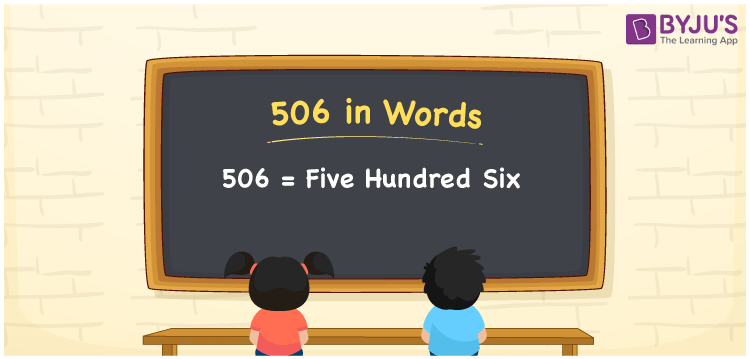# 506 in Words

506 in words is written as Five hundred six. In both the International System of Numerals and the Indian System of Numerals, 506 is written as Five hundred six. The number 506 is a Cardinal Number as it describes some quantity. For example, “There are 506 pages in that book”.

 506 in Words Five hundred six Five hundred six in Number 506

## 506 in English Words

We write 506 in English Words using the letters of the English alphabet. Therefore, we read 506 in English as “Five hundred six.”## How to Write 506 in Words?

To write 506 in words, we shall use the place value chart. In the place value chart, write 6 in the ones, 0 in the tens, and 5 in the hundreds. Now let us make a place value chart to write the number 506 in words.

 Hundreds Tens Ones 5 0 6

Thus, we can write the expanded form as

5 × Hundred + 0 × Ten + 6 × One

= 5 × 100 + 0 × 10 + 6 × 1

= 500 + 0 + 6

= 506

= Five hundred six.

506 is a natural number which is the successor of 505 and the predecessor of 507.

506 in words – Five hundred six

• Is 506 an odd number? – No
• Is 506 an even number? – Yes
• Is 506 a perfect square number? – No
• Is 506 a perfect cube number? – No
• Is 506 a prime number? – No
• Is 506 a composite number? – Yes

## Frequently Asked Questions on 506 in Words

Q1

### How to write 506 in words?

506 in words is written as Five hundred six.
Q2

### How to write 506 in words in the International and Indian System of Numerals?

In both the systems of numerals, 506 is written as Five hundred six.
Q3

### How to write 506 in a place value chart?

In the place value chart, write 6 in ones, 0 in tens, and then 5 in the hundreds place.